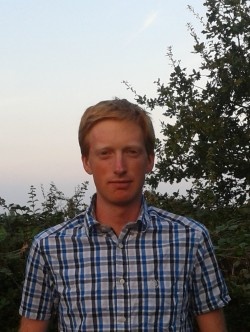# Jack ShottonI am an Assistant Professor in the Department of Mathematical Sciences at Durham University.

Previously I was an L. E. Dickson Instructor in the Department of Mathematics at the University of Chicago and a PhD student at Imperial College London under the supervision of Professor Toby Gee.

## Research

I am interested in number theory and representation theory, especially in Galois representations and arithmetic properties of automorphic forms.

### Papers.

1. Irreducible components of the moduli space of Langlands parameters.
Submitted.
pdf
2. On endomorphism algebras of Gelfand--Graev representations II.
with Tzu-Jan Li
To appear in Bulletin of the London Mathematical Society
pdf, journal.
3. Generic local deformation rings when $$l \neq p$$.
Compositio Mathematica, 158(4), 721-749 (2022).
pdf, journal.
4. Ihara's lemma for Shimura curves over totally real fields via patching.
with Jeffrey Manning.
Mathematische Annalen 379, 187-234 (2021).
pdf, journal.
5. The category of finitely presented mod p representations of $$GL_2(F)$$.
Documenta Mathematica 25 (2020), 143-157
pdf, journal
6. Local deformation rings for 2-adic deformation rings of $$G_{\mathbb{Q}_l}$$, $$l \neq 2$$.
Appendix to On crystabeline deformation rings of $$\mathrm{Gal}(\bar{\mathbb{Q}_p}/\mathbb{Q}_p)$$ by Yongquan Hu and Vytautas Paškūnas.
Mathematische Annalen 373 (2019), 421-487
pdf, journal.
7. The Breuil-Mézard conjecture when $$l \neq p$$.
Duke Mathematical Journal 167 (2018), no. 4, 603-678.
pdf, journal.
8. Local deformation rings for $$GL_2$$ and a Breuil-Mézard conjecture when $$l \neq p$$.
Algebra and Number Theory 10 (2016), no. 7, 1437-1475.
pdf, journal.
There is an error in the proof of Proposition 2.7 that is corrected in the pdf version here.
My thesis essentially contains the last two papers above.

### PhD Students

• Alex Milner (2023), "Invariant factors of elliptic curves", funded by the LMS.
• Patrick Creagh (2022), "Steinitz classes of CM elliptic curves".
• Dylan Johnston (2019), "Positivity and the weight part of Serre's conjecture", funded by the LMS.
• David Lin (2017), "The Kronecker-Weber theorem"

## Teaching.

• 2023-2024: Cryptography and Codes III (Michaelmas), Number Theory III (Epiphany).
• 2019-2023: Representation Theory IV. Lecture notes.
• 2018-2019: Cryptography and Codes III.

In 2023-2024 I will be offering two projects: Cyclotomic Polynomials at level III and Elliptic Curves at level IV.

Before coming to Durham I taught Introduction to Proof in Analysis and Linear Algebra, Honors Calculus, and Honors Basic Algebra at the University of Chicago.